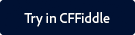Note:

You can find the CFFiddle demo of this function and other spreadsheet functions as part of a project that is shared with you.

Click the button below to launch CFFiddle.To copy the project in your workspace in CFFiddle, follow the steps below:

2. Navigate to the project in the left pane.
3. Once you make some changes in any cfm in the project, a pop up displays asking you to save the project.
4. Give the project a suitable name and click Save.

#### Description

Gets the Value for an Excel spreadsheet object cell.

#### Returns

A string containing the cell value.

#### Category

Microsoft Office Integration

#### Parameters

Parameter

Description

The Excel spreadsheet object from which to get the value.

row

The row number of the cell from which to get the formula.

column

The column number of the cell from which to get the formula.

#### Example

The following lines create an Excel spreadsheet object, set the value of the cell at row 3, column 5 - 365, gets the value and displays it:

 //Create a new Excel spreadsheet object. theSheet=SpreadsheetNew(); //Set the value of the cell at row 3 column 5. SpreadsheetSetCellValue(theSheet,365,3,5); //Get the value from the Excel spreadsheet object. theValue=SpreadsheetGetCellValue(theSheet,3,5); WriteOutput("The value of column 5 row 3 is: " & theValue);